Question

Answer Questions 7 through 13 using the diagram below. 7. Open Loop Transfer Function 8. Closed...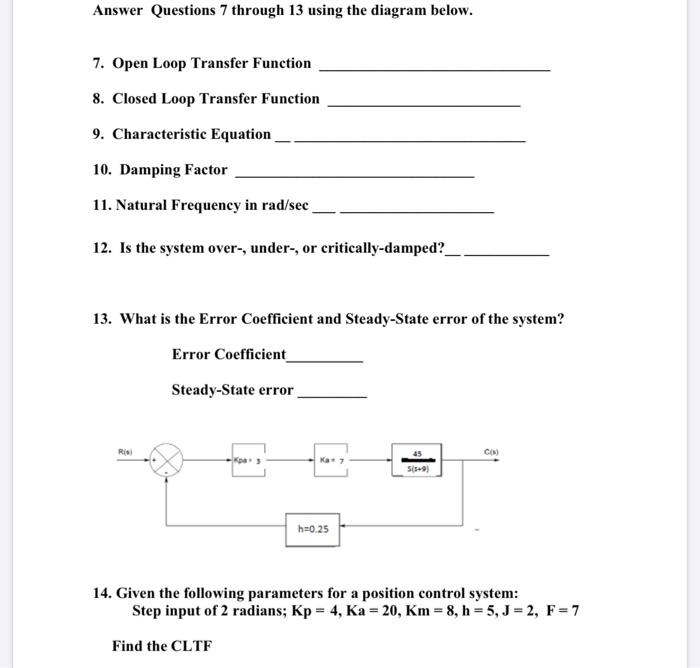Answer Questions 7 through 13 using the diagram below. 7. Open Loop Transfer Function 8. Closed Loop Transfer Function 9. Characteristic Equation 10. Damping Factor 11. Natural Frequency in rad/sec 12. Is the system over-, under-, or critically-damped?_ 13. What is the Error Coefficient and Steady-State error of the system? Error Coefficient Steady-State error h=0.25 14. Given the following parameters for a position control system: Step input of 2 radians; Kp = 4, Ka = 20, Km = 8, h = 5, J = 2, F = 7 Find the CLTE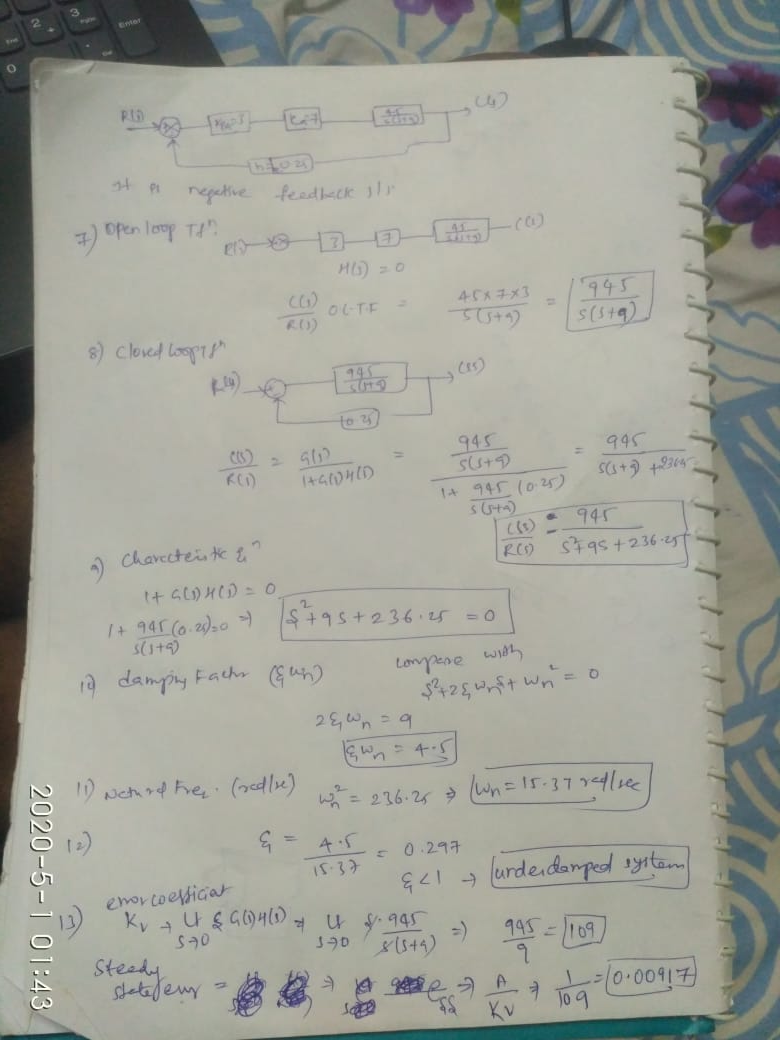Earn Coins

Coins can be redeemed for fabulous gifts.

Similar Homework Help Questions
• Consider the closed loop system defined by the following block diagram. a) Compute the transfer function...Consider the closed loop system defined by the following block diagram. a) Compute the transfer function E(s)/R(s). b) Determine the steady state error for a unit-step 1. Controller ant Itly Ro- +- HI- 4단Toy , c) d) e) reference input signal. Determine the steady state error response for a unit-ramp reference input signal. Determine the locations of the closed loop poles of the system. Select system parameters kp and ki in terms of k so that damping coefficient V2/2 and...

• show steps please 10 A second-order open-loop system with transfer function G(s) = is to be...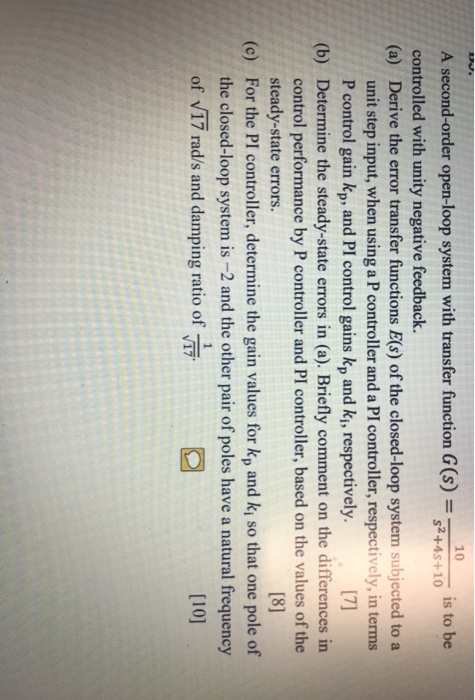show steps please 10 A second-order open-loop system with transfer function G(s) = is to be \$2+45+10 controlled with unity negative feedback. (a) Derive the error transfer functions E(s) of the closed-loop system subjected to a unit step input, when using a P controller and a PI controller, respectively, in terms P control gain kp, and PI control gains kp and ki, respectively.  (b) Determine the steady-state errors in (a). Briefly comment on the differences in control performance by...

• PD & PID controller design Consider a unity feedback system with open loop transfer function, G(s)...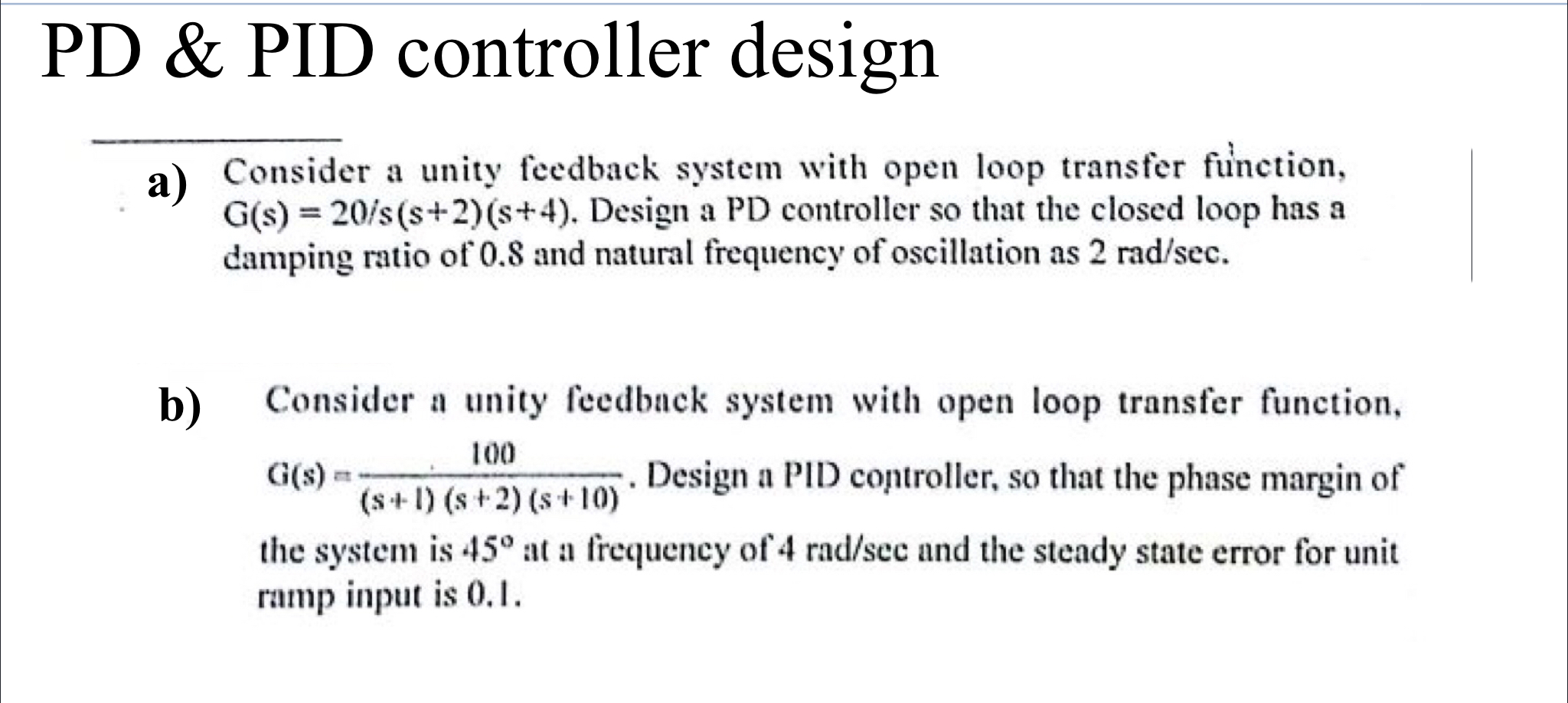PD & PID controller design Consider a unity feedback system with open loop transfer function, G(s) = 20/s(s+2)(8+4). Design a PD controller so that the closed loop has a damping ratio of 0.8 and natural frequency of oscillation as 2 rad/sec. b) 100 Consider a unity feedback system with open loop transfer function, aus. Design a PID controller, so that the phase margin of (S-1) (s + 2) (s+10) the system is 45° at a frequency of 4 rad/scc and...

• blem 5 (2000): The closed-loop system is given below. Controller El(s) ) (5% o) Find the system transfer function and discuss the range of Ko to make the stem stable assuming Kp-5. ) (5 %) Find t...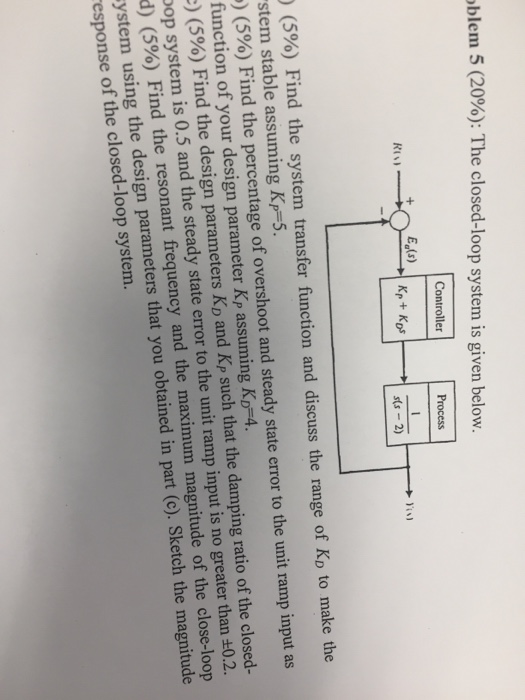blem 5 (2000): The closed-loop system is given below. Controller El(s) ) (5% o) Find the system transfer function and discuss the range of Ko to make the stem stable assuming Kp-5. ) (5 %) Find the percentage of overshoot and steady state error to the unit ramp input as function of your design parameter Kp assuming KD-4. :) (5%) Find the design parameters KD and Kp such that the damping ratio of the closed- pop system is 0.5 and...

•  Sketch the root locus for the unity feedback system whose open loop transfer function is K G(s) Draw the root lo...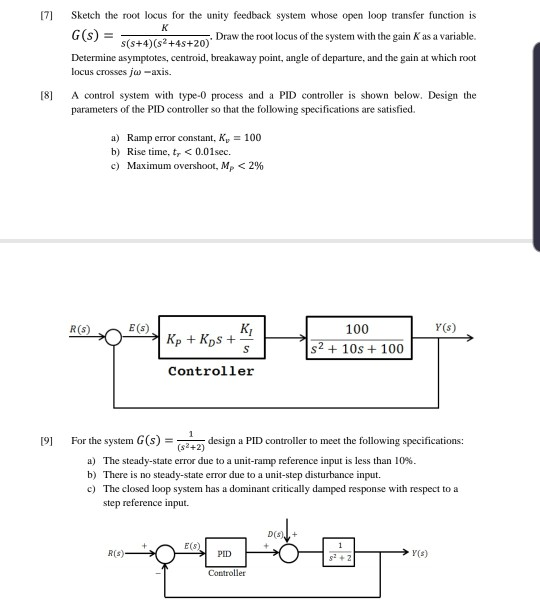Sketch the root locus for the unity feedback system whose open loop transfer function is K G(s) Draw the root locus of the system with the gain Kas a variable. s(s+4) (s2+4s+20) Determine asymptotes, centroid, breakaway point, angle of departure, and the gain at which root locus crosses ja-axis. A control system with type-0 process and a PID controller is shown below. Design the [8 parameters of the PID controller so that the following specifications are satisfied. =100 a)...

• b) Given a second order system with the following open loop transfer function where damping ratio,...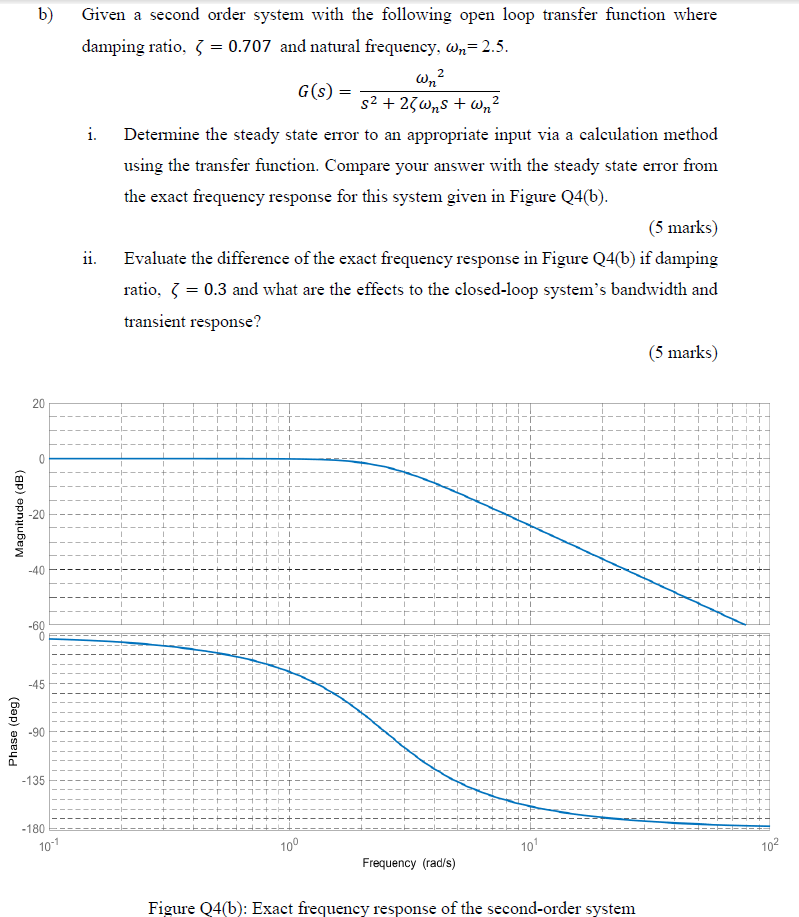b) Given a second order system with the following open loop transfer function where damping ratio, } = 0.707 and natural frequency, Wn= 2.5. wn? G(S) = S2 + 23wns +wn? i. Determine the steady state error to an appropriate input via a calculation method using the transfer function. Compare your answer with the steady state error from the exact frequency response for this system given in Figure Q4(b). (5 marks) ii. Evaluate the difference of the exact frequency response...

• Answer the following questions: К R(s) C(s) к, ST1 Find the closed loop transfer function from...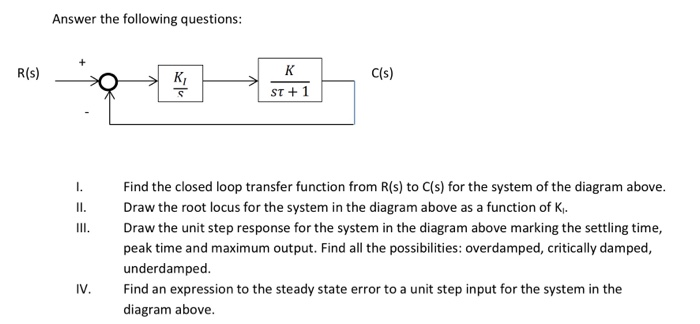Answer the following questions: К R(s) C(s) к, ST1 Find the closed loop transfer function from R(s) to C(s) for the system of the diagram above. Draw the root locus for the system in the diagram above as a function of K Draw the unit step response for the system in the diagram above marking the settling time, peak time and maximum output. Find all the possibilities: overdamped, critically damped, underdamped. Find an expression to the steady state error to...

• Question 6 The open-loop transfer function G(s) of a control system is given as G(8)- s(s+2)(s +5...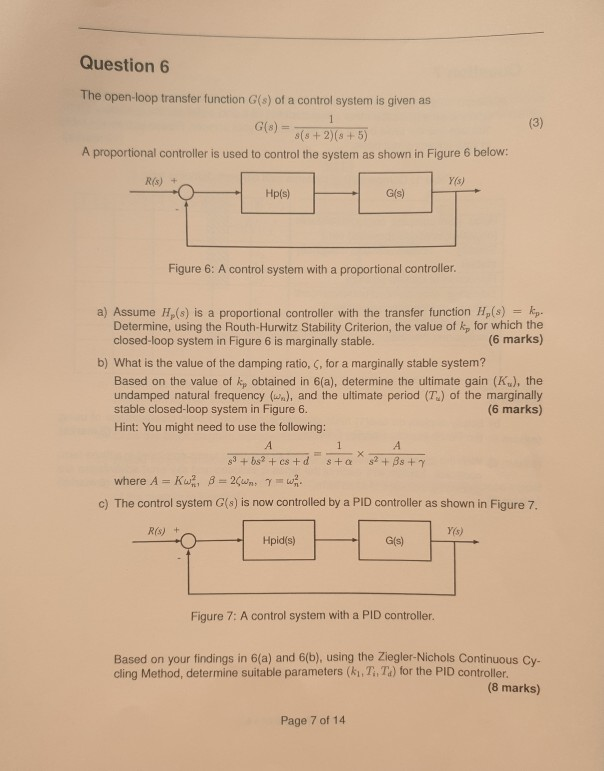Question 6 The open-loop transfer function G(s) of a control system is given as G(8)- s(s+2)(s +5) A proportional controller is used to control the system as shown in Figure 6 below: Y(s) R(s) + G(s) Figure 6: A control system with a proportional controller a) Assume Hp(s) is a proportional controller with the transfer function H,(s) kp. Determine, using the Routh-Hurwitz Stability Criterion, the value of kp for which the closed-loop system in Figure 6 is marginally stable. (6...

• 6. A second order differential equation d?x/dt+ 5 dx/dt+7x = 7y. State the undamped natural frequ...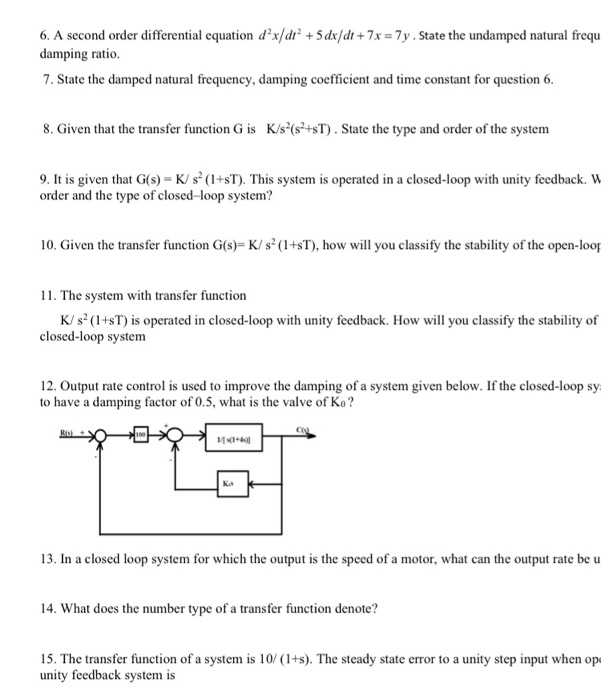6. A second order differential equation d?x/dt+ 5 dx/dt+7x = 7y. State the undamped natural frequ damping ratio. 7. State the damped natural frequency, damping coefficient and time constant for question 6. 8. Given that the transfer function G is K/s(s+sT). State the type and order of the system 9. It is given that G(s) = K/s (1+sT). This system is operated in a closed-loop with unity feedback. W order and the type of closed-loop system? 10. Given the transfer...

• Question 6. 30) Design a controller for the following open loop transfer function to achieve a da...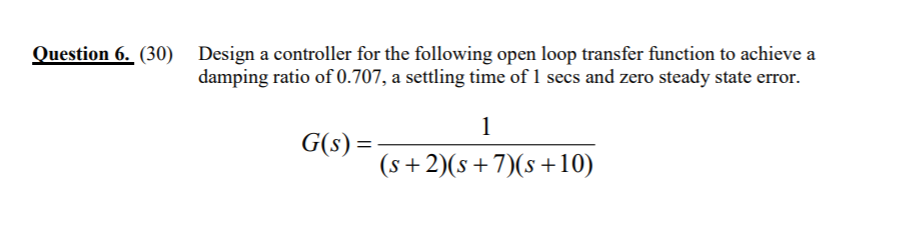Question 6. 30) Design a controller for the following open loop transfer function to achieve a damping ratio of 0.707, a settling time of 1 secs and zero steady state error G(s) s+2(s +7(s +10) Question 6. 30) Design a controller for the following open loop transfer function to achieve a damping ratio of 0.707, a settling time of 1 secs and zero steady state error G(s) s+2(s +7(s +10)